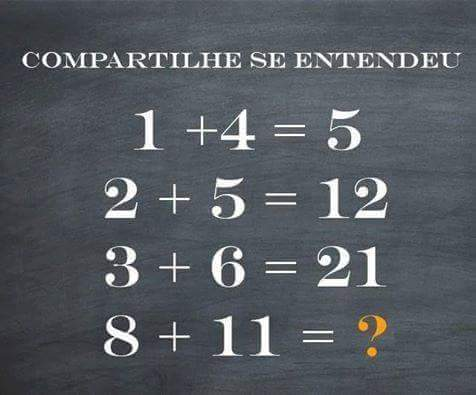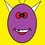# A math problem is able to have multiple right solutions

This "riddle" has raised more than one million comments on Facebook.What would be your answer to the question mark? The most common answers are 40 and 96... Does anyone could say why? Hence if it is given the first terms in a sequence and the sequence is not well defined, the next term is able has multiple right solutions, even infinite solutions. For instance, in the next problem Find the solution which I gave one solution, is able to have infinite answers, even the other answers given in the problem can be right... Just only have to take polynomials with two variables x,y which satisfies the conditions, for example,can you find a polynomial with variables x, y satisfying the first conditions and such that f(13,4) = 123 or f(10,9) = 123?... So my conclusion is this one: Can you post one riddle where different right solutions are valid?and why my "riddle" is able to have 40 or 96 as valid solutions?can you find other right solution and say why?Note by Guillermo Templado
5 years, 1 month ago

This discussion board is a place to discuss our Daily Challenges and the math and science related to those challenges. Explanations are more than just a solution — they should explain the steps and thinking strategies that you used to obtain the solution. Comments should further the discussion of math and science.

When posting on Brilliant:

• Use the emojis to react to an explanation, whether you're congratulating a job well done , or just really confused .
• Ask specific questions about the challenge or the steps in somebody's explanation. Well-posed questions can add a lot to the discussion, but posting "I don't understand!" doesn't help anyone.
• Try to contribute something new to the discussion, whether it is an extension, generalization or other idea related to the challenge.
• Stay on topic — we're all here to learn more about math and science, not to hear about your favorite get-rich-quick scheme or current world events.

MarkdownAppears as
*italics* or _italics_ italics
**bold** or __bold__ bold
- bulleted- list
• bulleted
• list
1. numbered2. list
1. numbered
2. list
Note: you must add a full line of space before and after lists for them to show up correctly
paragraph 1paragraph 2

paragraph 1

paragraph 2

[example link](https://brilliant.org)example link
> This is a quote
This is a quote
    # I indented these lines
# 4 spaces, and now they show
# up as a code block.

print "hello world"
# I indented these lines
# 4 spaces, and now they show
# up as a code block.

print "hello world"
MathAppears as
Remember to wrap math in $$ ... $$ or $ ... $ to ensure proper formatting.
2 \times 3 $2 \times 3$
2^{34} $2^{34}$
a_{i-1} $a_{i-1}$
\frac{2}{3} $\frac{2}{3}$
\sqrt{2} $\sqrt{2}$
\sum_{i=1}^3 $\sum_{i=1}^3$
\sin \theta $\sin \theta$
\boxed{123} $\boxed{123}$

## Comments

Sort by:

Top Newest

I think they expect 96 as the answer if they do this. $a+b=a×(b+1)$ But like @Nihar Mahajan said you can have $\infty$ solutions

- 5 years, 1 month ago

Log in to reply

The riddle has infinitely many solutions using LZOB or Lagrange interpolation, since the choices are not provided.

- 5 years, 1 month ago

Log in to reply

Why are you assuming the function is a polynomial? (just asking)

- 5 years, 1 month ago

Log in to reply

Its not wrong to assume a polynomial right?

- 5 years, 1 month ago

Log in to reply

I could be any arbitrary function of two variables for that matter as we've not been given that the function IS a polynomial.

- 5 years, 1 month ago

Log in to reply

So it can be $\infty$ if its a polynomial and if its some other function it can have finite or infinite. Therefore in general it can be said infinite

- 5 years, 1 month ago

Log in to reply

please, be calm. I can not answer to everybody....

- 5 years, 1 month ago

Log in to reply

Hey I was asking @Deeparaj Bhat. And it was a comment and not a question

- 5 years, 1 month ago

Log in to reply

ok, sorry, my apologies

- 5 years, 1 month ago

Log in to reply

please, be calm. I can not answer to everybody

- 5 years, 1 month ago

Log in to reply

How is the answer 96 or 40?

- 5 years, 1 month ago

Log in to reply

I got 96 but not 40.

- 5 years, 1 month ago

Log in to reply

ok, 96 can be got of this form, $\begin{cases}1 + 4 = 1 \cdot 4 + 1 = 5 \\ 2 + 5 = 2 \cdot 5 + 2 = 12 \\ 3 + 6 = 3 \cdot 6 + 3 = 21\end{cases}$ so I can say $8 + 11 = 8 \cdot 11 + 8 = 96$. Now, we are going to get 40,ok...please, be cal, wait my answer,haha..

- 5 years, 1 month ago

Log in to reply

Wait, I will wait. (LOL)

- 5 years, 1 month ago

Log in to reply

Now, we are going 40.. We can consider (1,4), (2,5), (3,6) and (8,11) to be the first terms of one sequence in $\mathbb{N}^2$ and $1 + 4 = 5$ and we can define for the next terms its sum added to the sum obtained before. Hence $2 + 5 = 7 + \text{ sum obtained before } = 7 + 5 = 12$ , now $3 + 6 = 9 + \text{ sum obtained before } = 9 + 12 = 21$ so $8 + 11 = 19 + \text{ sum obtained before } = 19 + 21 = 40$

- 5 years, 1 month ago

Log in to reply

Oh! I considered $8,11$ as the eighth term!

- 5 years, 1 month ago

Log in to reply

but, they can be considered the first terms in a sequence. Now I'm going with the Lagrange interpolation

- 5 years, 1 month ago

Log in to reply

please, be calm. I can not answer to everybody

- 5 years, 1 month ago

Log in to reply

please, be calm. I can not answer to everybody

- 5 years, 1 month ago

Log in to reply

We have $f(5,3) = 28, \space f(12,10) = 222 \space , f(9,1) = 810, \space f(4,2) = 26, \space f(8,3) = 511, f(10,1) = 911, \space f(7,3) = 410$. This problem using Lagrange interpolation is able to have $\infty$ answers. For example, I want furthemore $f(13,9) = 123.$ Is evrything allright so far? haha. Ok, I'm going to look for a polynomial with variables x,y and some constants a,b,c,d,e,f,g,h,i, ...fullfiling these conditions,(notice I'm going to repeat this factor (y -1) and (y - 3), I would not to do it, but I'm going to do it for clarity) so the polynomial has to be $\small f(x,y) = a(x -5)(y -3)(x -12)(y -10)(x - 9)(y -1)(x - 4)(y -2)(x - 8)(y -3)(x - 10)(y -1)(x - 7)(y - 3) +$ $\small + b(x -5)(y -3)(x -12)(y -10)(x - 9)(y -1)(x - 4)(y -2)(x - 8)(y -3)(x - 10)(y -1)(x - 13)(y -9) +$ $\small + c(x -5)(y -3)(x -12)(y -10)(x - 9)(y -1)(x - 4)(y -2)(x - 8)(y -3)(x - 7)(y - 3)(x -13)(y - 9) +$ $\small + d(x -5)(y -3)(x -12)(y -10)(x - 9)(y -1)(x - 4)(y -2)(x - 10)(y -1)(x - 7)(y - 3)(x - 13)(y - 9) +...$ and get the constants a,b ,c ,d e,f, g, h,... fulfilling the requisites... so this problem has $\infty$ right solutions... Ok, now I'm going to eat... Later I'll review the comments

- 5 years, 1 month ago

Log in to reply

Exactly, for the problem Find the solution you can use Lagrange interpolation with two variables, please wait a bit, and I'll give the answer

- 5 years, 1 month ago

Log in to reply

52

Assuming there is something fundamental operation that isn't explicit, just like the assumption that the last answer is also added to the next equation... Goes like this... The pattern is multiplying the second number secretly by a sequential while number, just like secretly having the last answer added to the next equation...

1+(4[×1])=5 2+(5[×2])=12 3+(6[×3])=21 8+(11[×4])=52

This makes all the answers correct.

- 3 years, 9 months ago

Log in to reply

## 8+(11[×4])=5

- 3 years, 9 months ago

Log in to reply

The answer is 40. :P
In this riddle the answer of the previous statement is added to the terms of the original statement.
First statement starts with 1 + 4 = $\color{#D61F06}{\text{5}}$.
Second statement:- $\color{#D61F06}{\text{5}}$ + 2 + 5 = $\color{#3D99F6}{\text {12}}$.
Third statement:- $\color{#3D99F6}{\text {12}}$ + 3 + 6 = $\color{#20A900}{\text {21}}$.
Fourth statement should be $\color{#20A900}{\text {21}}$ + 8 + 11 = $\boxed{40}$.

- 5 years, 1 month ago

Log in to reply

## 3+(6[×3])=21

8+(11[×4])=52

- 3 years, 9 months ago

Log in to reply

That was supposed to be a clarification of my own post... Sorry. 'Doing this from my phone while walking to the store.

- 3 years, 9 months ago

Log in to reply

×

Problem Loading...

Note Loading...

Set Loading...